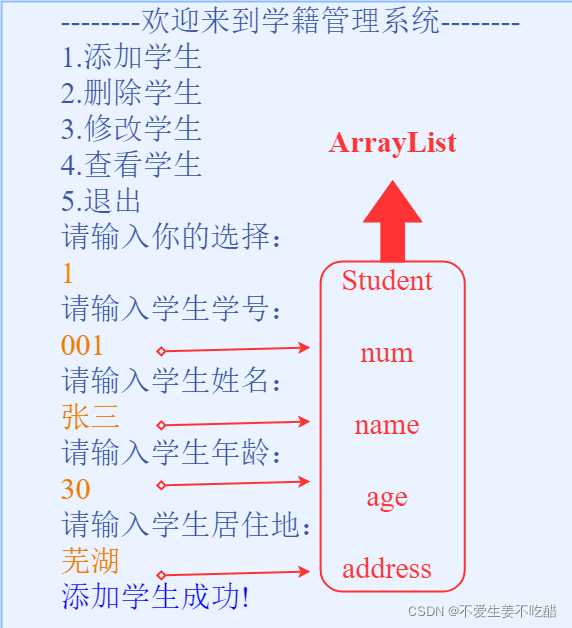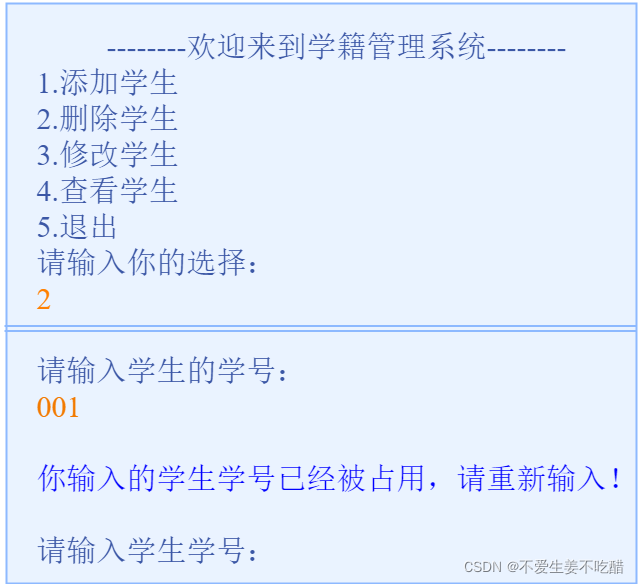﻿ Java超详细教你写一个学籍管理系统案例_java_脚本之家
java# Java超详细教你写一个学籍管理系统案例

## 一、学籍管理系统功能介绍

### 2.学籍管理系统的主要功能图示### 3.代码编写的思路

• 3.1 定义学生类
• 3.2 主界面的代码编写
• 3.3 添加学生的代码编写
• 3.4 查看学生的代码编写
• 3.5 删除学生的代码编写
• 3.6 修改学生的代码编写

## 二、定义学生类

Student

• 学号：num
• 姓名：name
• 年龄：age

### 5.代码示例

```public class Student {
private String num;//学生学号
private String name;//学生姓名
private String age;//学生年龄

public Student() {
}//无参构造

public Student(String num, String name, String age, String address) {
this.num = num;
this.name = name;
this.age = age;
}//带四个参数的构造

public String getNum() {
return num;
}

public void setNum(String num) {
this.num = num;
}

public String getName() {
return name;
}

public void setName(String name) {
this.name = name;
}

public String getAge() {
return age;
}

public void setAge(String age) {
this.age = age;
}

}

}```

## 三、主界面的代码编写

### 1.思路

• 新建一个主类（Studentdemo）
• 用输出语句完成主界面的编写
• `Scanner`实现键盘录入数据
• `switch`语句完成操作的选择
• 用循环完成再次回到主界面
• 循环之中包含添加、查找、删除、修改的方法

### 2.代码示例

```public class Studentdemo {
public static void main(String[] args){
ArrayList<Student> array=new ArrayList<>();
while(true) {
System.out.println("--------学生管理系统---------");
System.out.println("1.添加学生");
System.out.println("2.删除学生");
System.out.println("3.修改学生");
System.out.println("4.查看所有学生");
System.out.println("5.退出");
System.out.println("请选择你需要的操作：");

Scanner sc = new Scanner(System.in);
String line = sc.nextLine();

switch (line) {
case "1":
//**此处调用“添加学生方法”**
break;
case "2":
//**此处调用“删除学生方法”**
break;
case "3":
//**此处调用“修改学生方法”**
break;
case "4":
//**此处调用“查找学生方法”**
break;
case "5":
System.out.println("谢谢使用！");
System.exit(0);
}
}
}
}```

## 四、系统功能方法的实现

### 1.添加学生信息的代码编写

#### 1.1 思路

• 显示提示信息
• 键盘录入学生对象信息所需要的数据
• 创建学生对象，将录入的数据赋值给学生对象的成员变量
• 将学生对象添加到集合中
• 给出添加成功提示

#### 1.2 添加学生信息的方法说明图#### 1.3 代码示例

```    public static void Addstudent(ArrayList<Student> array){
// 定义一个方法用于添加学生；
Scanner sc=new Scanner(System.in);

System.out.print("请输入学生学号：");
String num=sc.nextLine();
System.out.print("请输入学生姓名：");
String name=sc.nextLine();
System.out.print("请输入学生年龄：");
String age=sc.nextLine();
System.out.print("请输入学生地址：");

//创建学生对象，将键盘录入的数据赋值给学生对象的成员变量
Student s=new Student();
s.setNum(num);
s.setName(name);
s.setAge(age);

//将学生对象添加到集合中

//给出添加成功提示
System.out.println("添加学生成功！");
}```

### 2.查看学生信息的代码编写

#### 2.1 思路

• 显示表头信息
• 将集合中数据取出按照对应格式显示学生信息

#### 2.2 查看学生信息的方法说明图#### 2.3 代码示例

```    // 定义一个方法，用于查看学生信息；
public static void Findallstudent(ArrayList<Student> array){
if(array.size()==0){
System.out.println("无信息！");
return;
}
// 显示表头信息
// 将集合中数据取出按照对应格式显示学生信息
for(int i=0;i<array.size();i++){
Student s=array.get(i);
System.out.println("学号："+s.getNum());
System.out.println("姓名："+s.getName());
System.out.println("年龄："+s.getAge());
System.out.println("  ");
}
}```

### 3.删除学生信息的代码编写

#### 3.1 思路

• 显示提示信息
• 键盘录入要删除的学生学号
• 遍历集合将对应学生对象从集合中删除
• 给出删除成功提示

#### 3.2 删除学生信息的方法说明图#### 3.3 代码示例

```  // 定义一个方法，用于删除学生信息；
public static void Deletestudent(ArrayList<Student> array){
// 键盘录入要删除的学生信息，显示提示信息
Scanner sc=new Scanner(System.in);
System.out.println("请输入要删除学生的学号：");
String num=sc.nextLine();

int index = -1;

// 遍历集合将对应学生对象从集合中删除
for(int i=0;i<array.size();i++){
Student s=array.get(i);
if(s.getNum().equals(num)){
index = i;
break;
}
}

if(index == -1){
System.out.println("学号有误！");
}else{
array.remove(index);
System.out.println("删除学生信息成功！");
}
}
```

### 4.修改学生的代码编写

#### 4.1 思路

• 显示提示信息
• 键盘录入要修改的学生学号
• 遍历集合修改对应的学生信息
• 给出修改成功提示

#### 4.2 修改学生信息的方法说明图#### 4.3 代码示例

```    // 定义一个方法，用于修改学生信息；
// 键盘录入选择修改的学生信息，显示提示信息
Scanner sc=new Scanner(System.in);
System.out.print("请输入要修改的学生学号：");
String num=sc.nextLine();

// 键盘录入要修改的学生信息
System.out.print("请输入修改后的学生姓名");
String name=sc.nextLine();
System.out.print("请输入修改后的学生年龄");
String age=sc.nextLine();
System.out.print("请输入修改后的学生地址");

//创建学生对象
Student s=new Student();
s.setNum(num);
s.setName(name);
s.setAge(age);
//遍历集合修改对应的学生信息
for(int i=0;i<array.size();i++){
Student student=array.get(i);
if(student.getNum().equals(num)){
array.set(i,s);
break;
}
}
//修改成功提示
System.out.println("修改成功！");
}
```

### 5.学号重复问题

#### 5.1 思路

• 如果与集合中的某一个学生学号相同，返回true
• 如果都不相同，返回false;

• 如果返回true，提示重新输入学号
• 如果返回false，正常添加学生对象

#### 5.2 学号被占用的方法说明图#### 5.3 代码示例

```     // 定义一个方法，对学号是否被使用进行判断；
public static boolean Isused(ArrayList<Student> array,String num){
// 如果与集合中的某一个学生学号相同，返回true
// 如果都不相同，返回false;
boolean flag =false;

for(int i=0;i<array.size();i++){
Student s=array.get(i);
if(s.getNum().equals(num)){
flag = true;
break;
}
}
return flag;
// 在添加学生录入学号后调用该方法
// 如果返回true，提示重新输入学号
// 如果返回false，正常添加学生对象
}
```

#### 5.4 代码示例

```     public static void Addstudent(ArrayList<Student> array){
// 定义一个方法用于添加学生；
Scanner sc=new Scanner(System.in);

String num;

//为了让程序回到这里，我们使用循环实现
while(true){
System.out.print("请输入学生学号：");
num = sc.nextLine();

boolean flag=Isused(array,num);
if(flag){
System.out.println("你输入的学号已经被使用，请重新输入：");
}else{
break;
}
}

System.out.print("请输入学生姓名：");
String name=sc.nextLine();
System.out.print("请输入学生年龄：");
String age=sc.nextLine();
System.out.print("请输入学生地址：");

//创建学生对象，将键盘录入的数据赋值给学生对象的成员变量
Student s=new Student();
s.setNum(num);
s.setName(name);
s.setAge(age);

//将学生对象添加到集合中

//给出添加成功提示
System.out.println("添加学生成功！");
}
```

（注意：如果之前有调用了标题4中的方法，应先删去后在调用改进后的添加学生信息方法！）